Next:7.2.3 All Pairs Shortest Paths Problem: Floyd's AlgorithmUp:7.2 Shortest Paths ProblemPrevious:7.2.1 Single Source Shortest Paths Problem: Dijkstra's Algorithm

## 7.2.2 Dynamic Programming Algorithm

REF.
Richard Bellman. Dynamic Programming. Princeton University Press, 1957.
REF.
Richard Bellman. On a routing problem. Quarterly of Applied Mathematics, Volume 16, Number 1, pp. 87-90, 1958.
Consider a directed acyclic graph (digraph without cycles) with nonnegative weights on the directed arcs.

Given a destination vertex z, the problem is to find a shortest cost path from each vertex of the digraph. See Figure 7.6.

Let

 C(i, j) =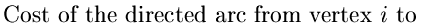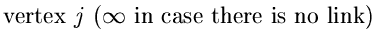(7.8) J(i) =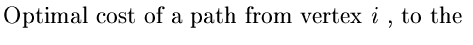(7.9)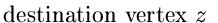J(i) Satisfies :

J(z) = 0
and if the optimal path from i to z traverses the link (i, j) then
J(i) = C(i, j) + J(j)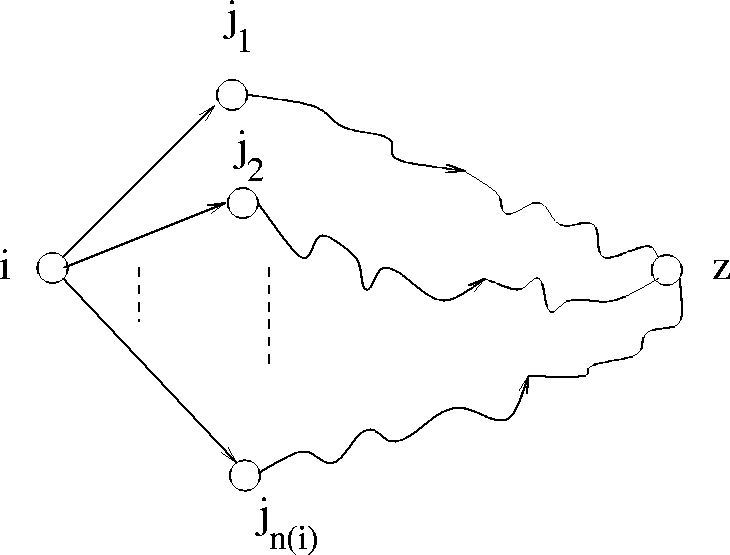Suppose that for each vertex j such that the link (i, j) exists, the optimal cost J(j) is known. Then, the principle of DP immediately implies:

J(i) =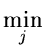[C(i, j) + J(j)]
A DP algorithm based on the above observations:
1.
Set J(z) = 0. At this point, this is the only node whose cost has been computed.
2.
Consider vertices i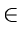V such that
• J(i) has not yet been found
• for each vertex j such that a link (i, j) exists, J(j) is already computed
Assign J(i) according to

J(i) =[C(i, j) + J(j)]

3.
Repeat Step 2 until all vertices have their optimal costs computed.
Example: Consider the digraph in Figure 7.7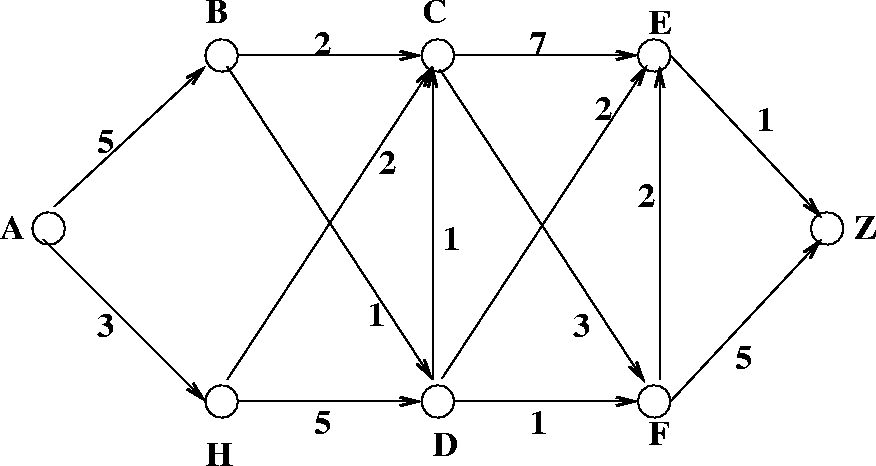Step 1.
J(Z) = 0
Step 2.
E is the only vertex such that J(j) is known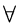I>j such that C(E, j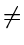0
J(E) = C(E, Z) + J(Z) = 1
Step 3.
Select F.
 J(F) = min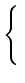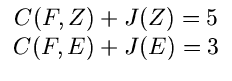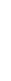(7.10) = min(5, 3) = 3

This yields the optimal path F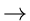EZ.

Step 4.
Select C.
 J(C) = min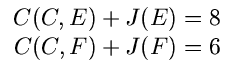(7.11) = min(8, 6) = 6

This yields the optimal path CFEZ.

Step 5.
Select D
J(D) = min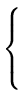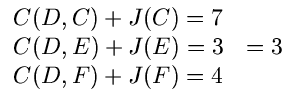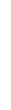This yields the optimal path DEZ.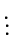Eventually, we get all the optimal paths and all the optimal costs.

Complexity of the Algorithm
• It is easy to see that the algorithm has a worst case complexity of O(n2), where n is the number of vertices.
• Limitation of the algorithm
• doesn't work if there are cycles in the digraph.Next:7.2.3 All Pairs Shortest Paths Problem: Floyd's AlgorithmUp:7.2 Shortest Paths ProblemPrevious:7.2.1 Single Source Shortest Paths Problem: Dijkstra's Algorithm
eEL,CSA_Dept,IISc,Bangalore